# Speech Recognition Hidden Markov Models for Speech Recognition

• Slides: 40Speech Recognition Hidden Markov Models for Speech Recognition Veton Këpuska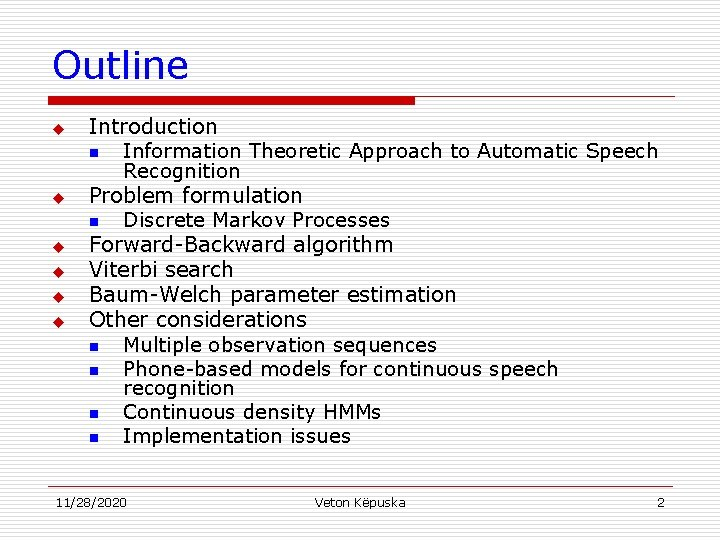Outline u u u Introduction n Information Theoretic Approach to Automatic Speech Recognition Problem formulation n Discrete Markov Processes Forward-Backward algorithm Viterbi search Baum-Welch parameter estimation Other considerations n Multiple observation sequences n Phone-based models for continuous speech recognition n Continuous density HMMs n Implementation issues 11/28/2020 Veton Këpuska 2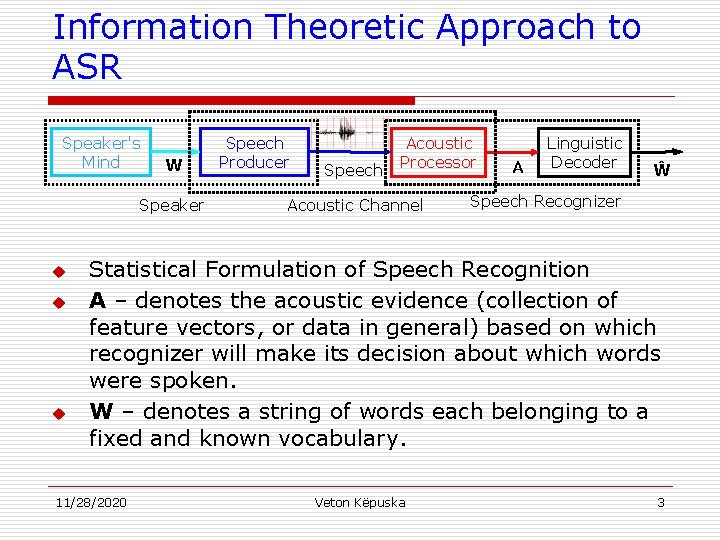Information Theoretic Approach to ASR Speaker's Mind W Speaker u u u Speech Producer Speech Acoustic Processor Acoustic Channel A Linguistic Decoder Ŵ Speech Recognizer Statistical Formulation of Speech Recognition A – denotes the acoustic evidence (collection of feature vectors, or data in general) based on which recognizer will make its decision about which words were spoken. W – denotes a string of words each belonging to a fixed and known vocabulary. 11/28/2020 Veton Këpuska 3Information Theoretic Approach to ASR n n Assume that A is a sequence of symbols taken from some alphabet A. W – denotes a string of n words each belonging to a fixed and known vocabulary V. 11/28/2020 Veton Këpuska 4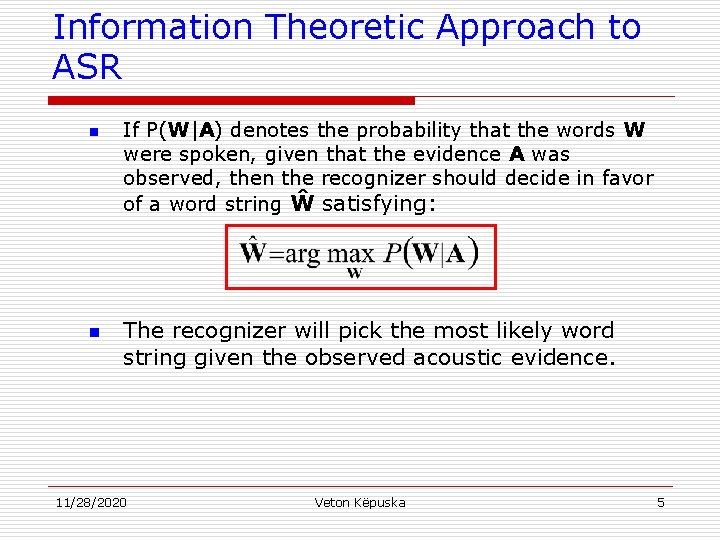Information Theoretic Approach to ASR n n If P(W|A) denotes the probability that the words W were spoken, given that the evidence A was observed, then the recognizer should decide in favor of a word string Ŵ satisfying: The recognizer will pick the most likely word string given the observed acoustic evidence. 11/28/2020 Veton Këpuska 5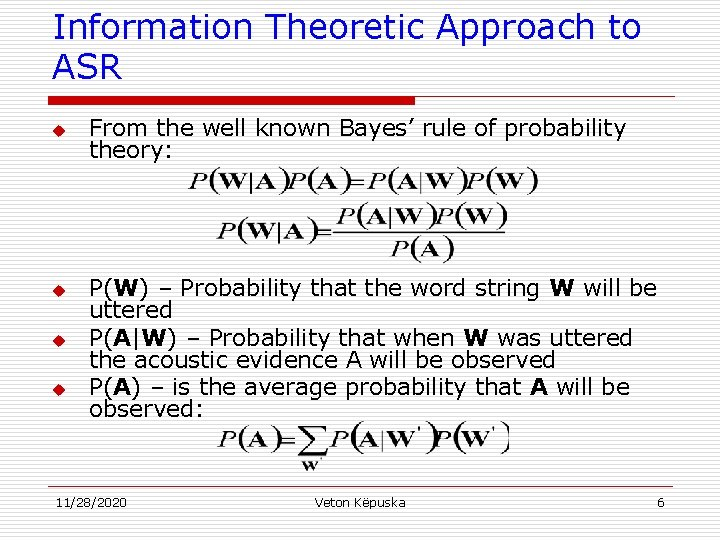Information Theoretic Approach to ASR u u From the well known Bayes’ rule of probability theory: P(W) – Probability that the word string W will be uttered P(A|W) – Probability that when W was uttered the acoustic evidence A will be observed P(A) – is the average probability that A will be observed: 11/28/2020 Veton Këpuska 6Information Theoretic Approach to ASR u u Since Maximization in: Is carried out with the variable A fixed (e. g. , there is not other acoustic data save the one we are give), it follows from Baye’s rule that the recognizer’s aim is to find the word string maximizes the product 11/28/2020 Ŵ that P(A|W)P(W), that is Veton Këpuska 7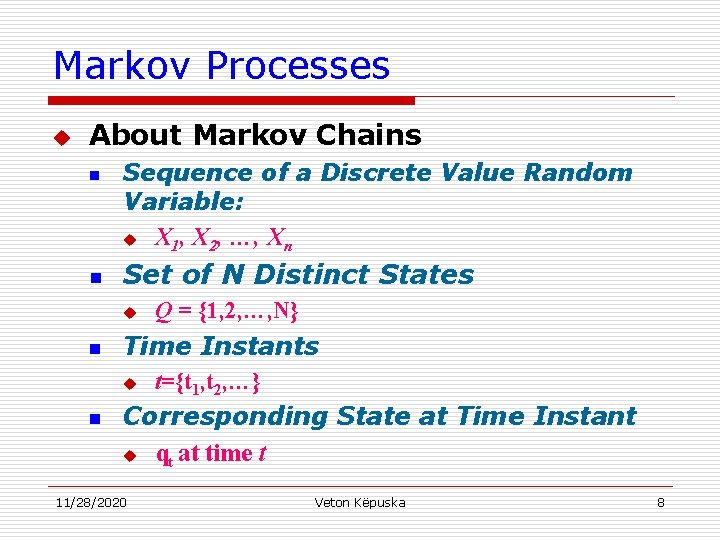Markov Processes u About Markov Chains n n Sequence of a Discrete Value Random Variable: u X 1, X 2, …, Xn Set of N Distinct States u n n Q = {1, 2, …, N} Time Instants u t={t 1, t 2, …} Corresponding State at Time Instant u qt at time t 11/28/2020 Veton Këpuska 8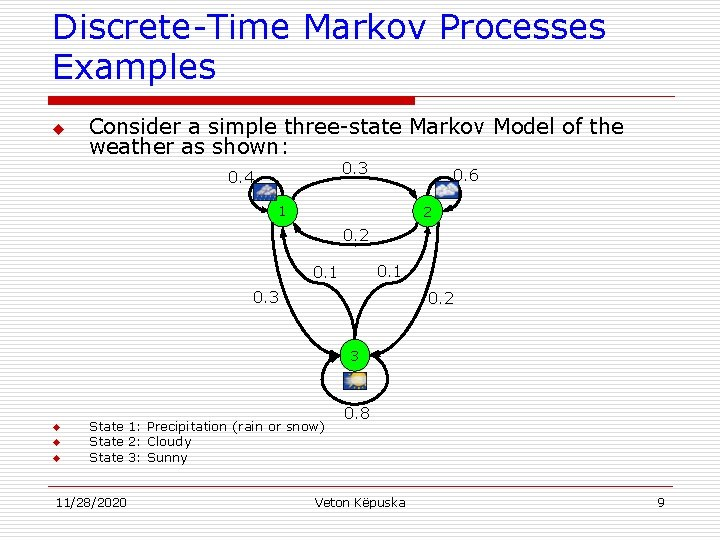Discrete-Time Markov Processes Examples u Consider a simple three-state Markov Model of the weather as shown: 0. 3 0. 4 0. 6 1 2 0. 1 0. 3 0. 2 3 u u u State 1: Precipitation (rain or snow) State 2: Cloudy State 3: Sunny 11/28/2020 0. 8 Veton Këpuska 9Discrete-Time Markov Processes Examples u u Matrix of state transition probabilities: Given the model in the previous slide we can now ask (and answer) several interesting questions about weather patterns over time. 11/28/2020 Veton Këpuska 10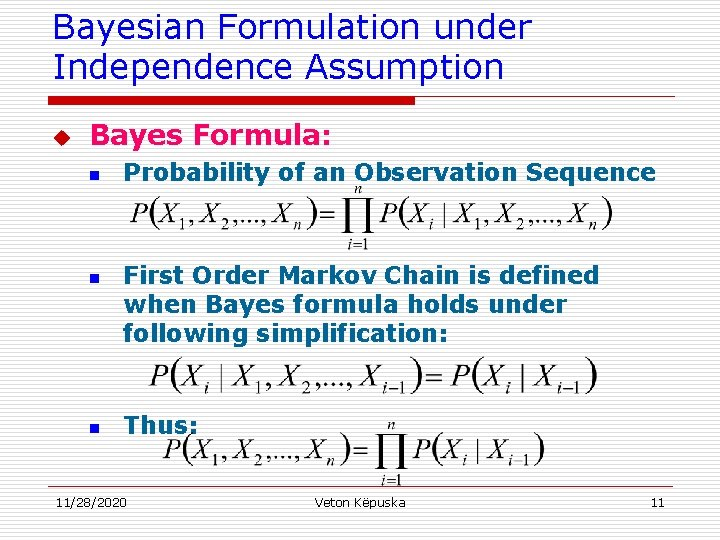Bayesian Formulation under Independence Assumption u Bayes Formula: n n n Probability of an Observation Sequence First Order Markov Chain is defined when Bayes formula holds under following simplification: Thus: 11/28/2020 Veton Këpuska 11Markov Chain u Random Process has the simplest memory in First Order Markov Chain: n n The value at time ti depends only on the value at the preceding time ti-1 and on Nothing that went on before 11/28/2020 Veton Këpuska 12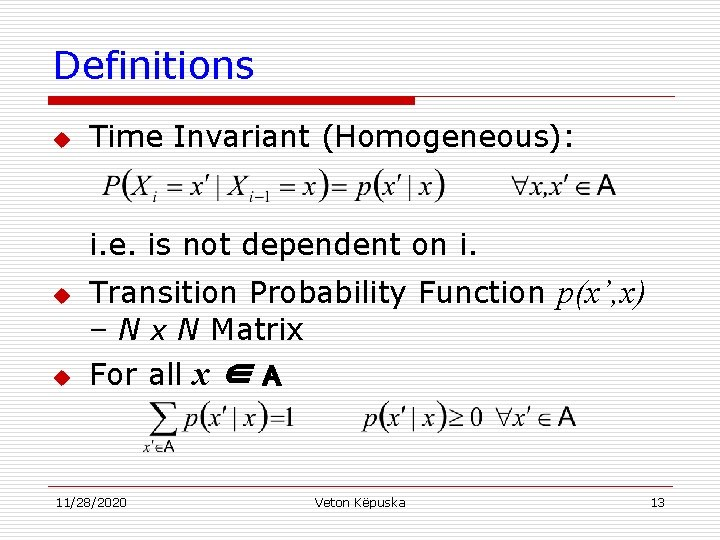Definitions u Time Invariant (Homogeneous): i. e. is not dependent on i. u u Transition Probability Function p(x’, x) – N x N Matrix For all x ∈ A 11/28/2020 Veton Këpuska 13Definitions u Definition of State Transition Probability: n aij = P(qt+1=sj|qt=si), 11/28/2020 Veton Këpuska 1 ≤ i, j ≤ N 14Discrete-Time Markov Processes Examples u Problem 1: What is the probability (according to the model) that the weather for eight consecutive days is “sun-sunsun-rain-sun-cloudy-sun”? Solution: n Define the observation sequence, O, as: n u Day 1 O = ( sunny, O=( 3, n 2 3 4 5 sunny, rain, 3, 1, 6 7 sunny, cloudy, 3, 8 sunny ) 2, 3) Want to calculate P(O|Model), the probability of observation sequence O, given the model of previous slide. Given that: 11/28/2020 Veton Këpuska 15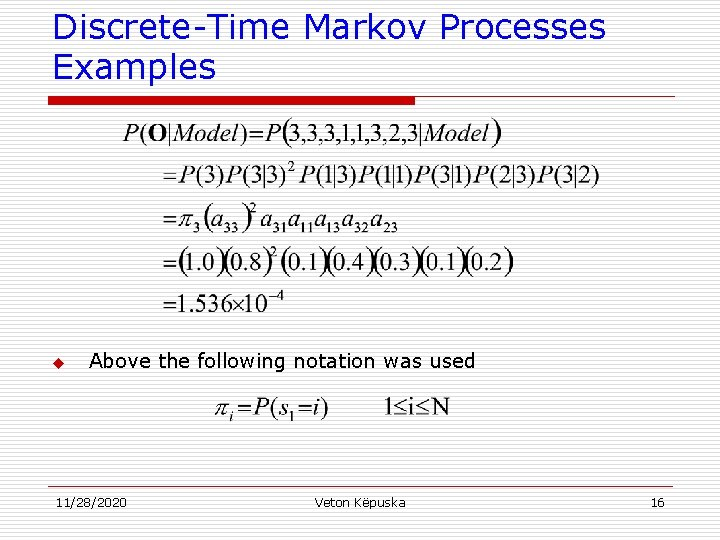Discrete-Time Markov Processes Examples u Above the following notation was used 11/28/2020 Veton Këpuska 16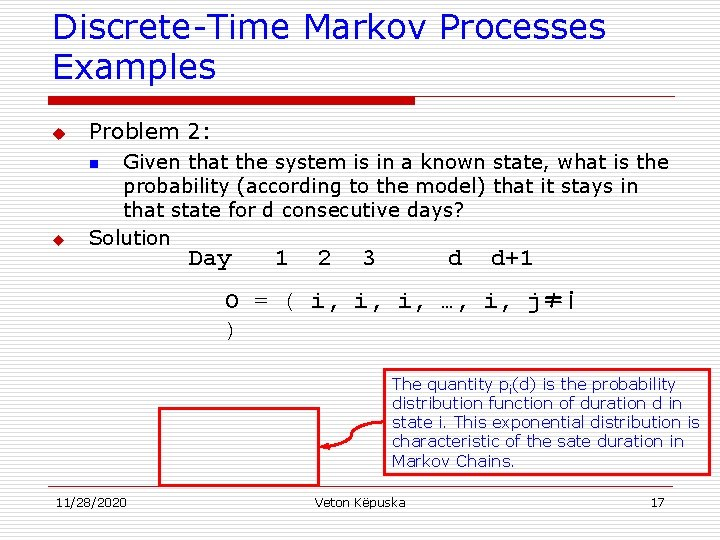Discrete-Time Markov Processes Examples u Problem 2: u Given that the system is in a known state, what is the probability (according to the model) that it stays in that state for d consecutive days? Solution n Day 1 2 3 d d+1 O = ( i, i, i, …, i, j≠i ) The quantity pi(d) is the probability distribution function of duration d in state i. This exponential distribution is characteristic of the sate duration in Markov Chains. 11/28/2020 Veton Këpuska 17Discrete-Time Markov Processes Examples n n Expected number of observations (duration) in a state conditioned on starting in that state can be computed as Thus, according to the model, the expected number of consecutive days of u u u Sunny weather: 1/0. 2=5 Cloudy weather: 2. 5 Exercise Problem: Derive the above formula or directly mean of pi(d) Rainy weather: 1. 67 Hint: 11/28/2020 Veton Këpuska 18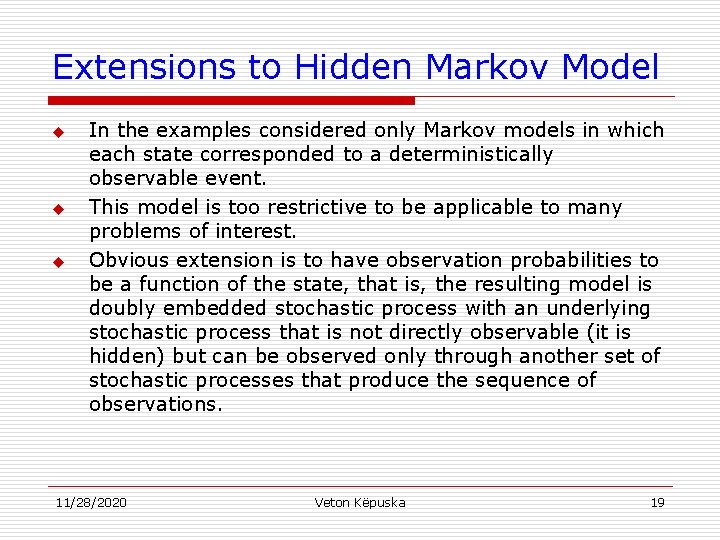Extensions to Hidden Markov Model u u u In the examples considered only Markov models in which each state corresponded to a deterministically observable event. This model is too restrictive to be applicable to many problems of interest. Obvious extension is to have observation probabilities to be a function of the state, that is, the resulting model is doubly embedded stochastic process with an underlying stochastic process that is not directly observable (it is hidden) but can be observed only through another set of stochastic processes that produce the sequence of observations. 11/28/2020 Veton Këpuska 19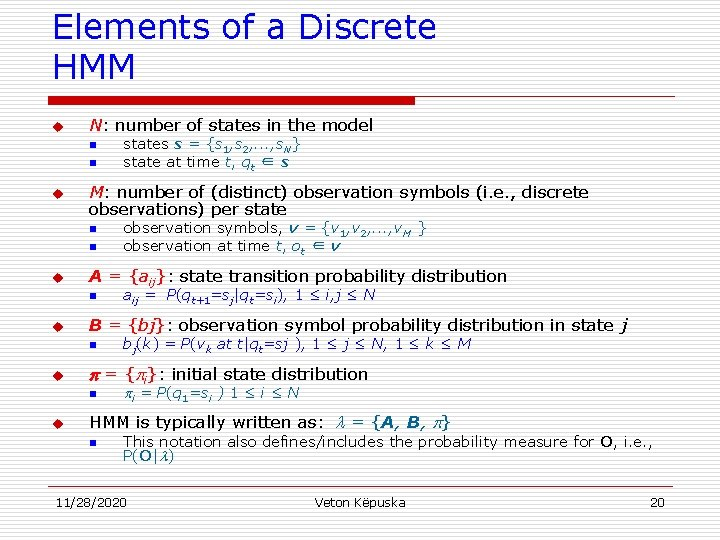Elements of a Discrete HMM u N: number of states in the model n n u M: number of (distinct) observation symbols (i. e. , discrete observations) per state n n u bj(k) = P(vk at t|qt=sj ), 1 ≤ j ≤ N, 1 ≤ k ≤ M = { i}: initial state distribution n u aij = P(qt+1=sj|qt=si), 1 ≤ i, j ≤ N B = {bj}: observation symbol probability distribution in state j n u observation symbols, v = {v 1, v 2, . . . , v. M } observation at time t, ot ∈ v A = {aij}: state transition probability distribution n u states s = {s 1, s 2, . . . , s. N} state at time t, qt ∈ s i = P(q 1=si ) 1 ≤ i ≤ N HMM is typically written as: = {A, B, } n This notation also defines/includes the probability measure for O, i. e. , P(O| ) 11/28/2020 Veton Këpuska 20State View of Markov Chain u u u Finite State Process Transitions between states specified by p(x’, x) For a small alphabet A Markov Chain can be specified by a diagram as in next figure: p(1|3) p(1|1) 1 p(3|1) p(2|3) p(2|1) 3 p(3|2) 2 Example of Three State Markov Chain 11/28/2020 Veton Këpuska 21One-Step Memory of Markov Chain u u u Does not restrict in modeling processes of arbitrary complexity: Define Random Variable Xi: Then the Z-sequence specifies the X-sequence, and vice versa The X process is a Markov Chain for which formula holds. Resulting space is very large and the Z process can be characterized directly in a much simpler way. 11/28/2020 Veton Këpuska 22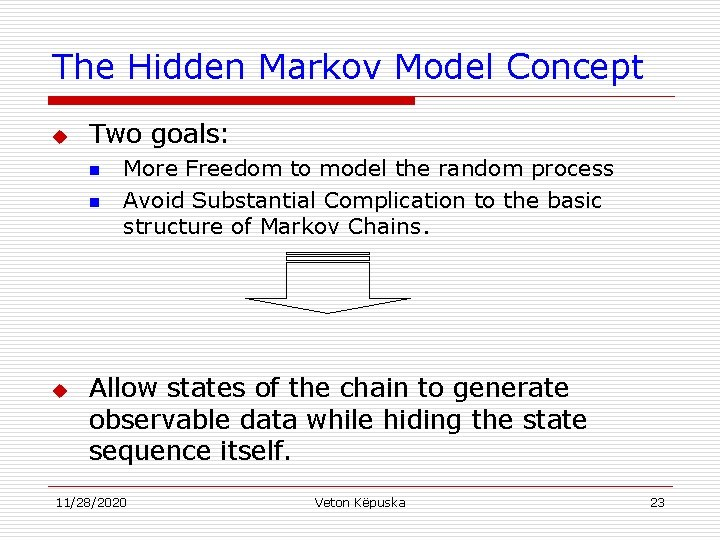The Hidden Markov Model Concept u Two goals: n n u More Freedom to model the random process Avoid Substantial Complication to the basic structure of Markov Chains. Allow states of the chain to generate observable data while hiding the state sequence itself. 11/28/2020 Veton Këpuska 23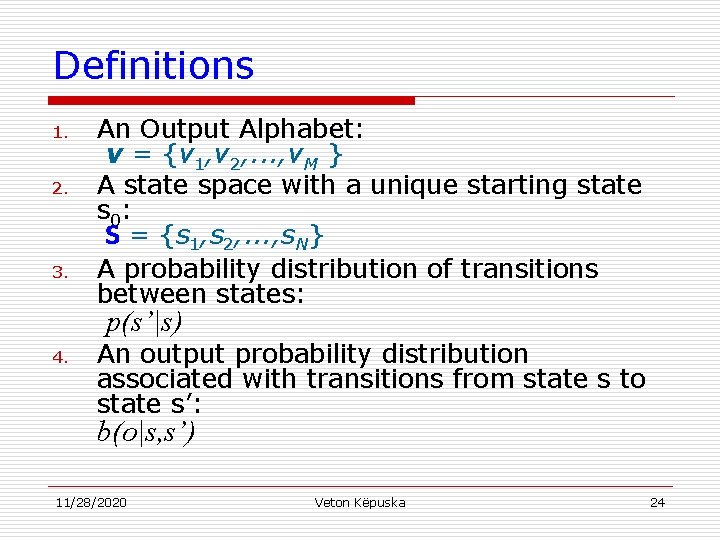Definitions 1. 2. 3. An Output Alphabet: v = {v 1, v 2, . . . , v. M } A state space with a unique starting state s 0: S = {s 1, s 2, . . . , s. N} A probability distribution of transitions between states: p(s’|s) 4. An output probability distribution associated with transitions from state s to state s’: b(o|s, s’) 11/28/2020 Veton Këpuska 24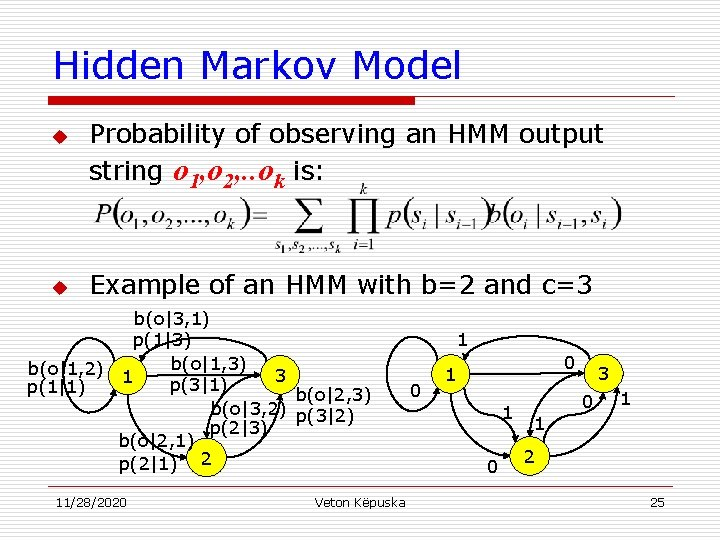Hidden Markov Model u u Probability of observing an HMM output string o 1, o 2, . . ok is: Example of an HMM with b=2 and c=3 b(o|3, 1) p(1|3) b(o|1, 2) 1 3 p(3|1) p(1|1) b(o|2, 3) b(o|3, 2) p(3|2) p(2|3) b(o|2, 1) p(2|1) 2 11/28/2020 Veton Këpuska 1 0 0 1 1 0 1 3 0 1 2 25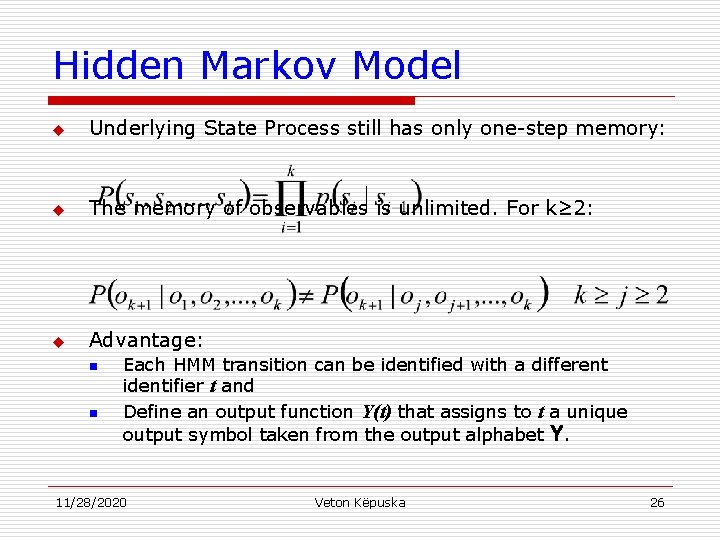Hidden Markov Model u Underlying State Process still has only one-step memory: u The memory of observables is unlimited. For k≥ 2: u Advantage: n n Each HMM transition can be identified with a different identifier t and Define an output function Y(t) that assigns to t a unique output symbol taken from the output alphabet Y. 11/28/2020 Veton Këpuska 26Hidden Markov Model u For a transition t denote: n L(t) – source state n R(t) – target state n p(t) – probability that the state is exited via the transition n t Thus for all s ∈ S 11/28/2020 Veton Këpuska 27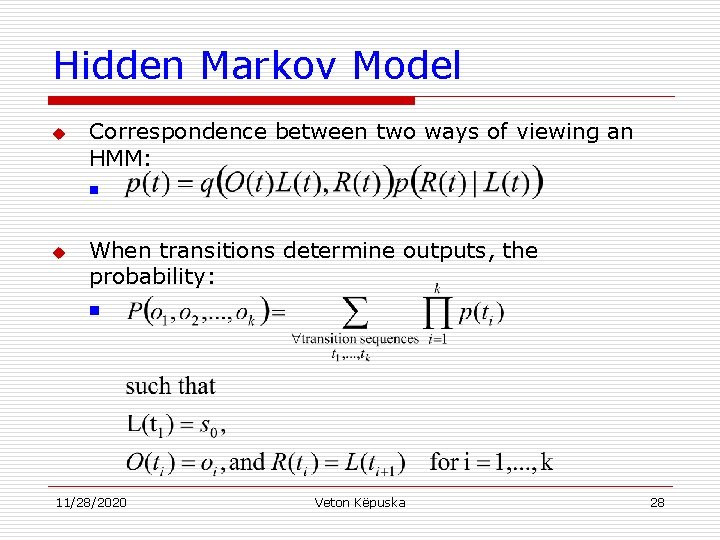Hidden Markov Model u Correspondence between two ways of viewing an HMM: n u When transitions determine outputs, the probability: n 11/28/2020 Veton Këpuska 28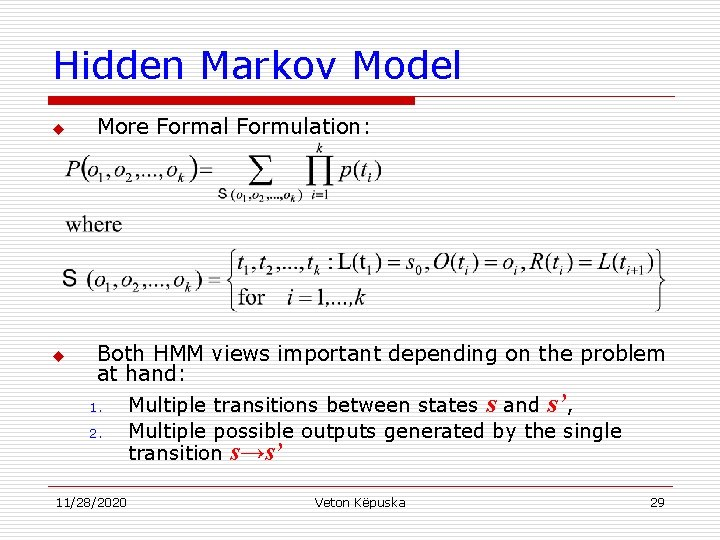Hidden Markov Model u u More Formal Formulation: Both HMM views important depending on the problem at hand: 1. Multiple transitions between states s and s’, 2. Multiple possible outputs generated by the single transition s→s’ 11/28/2020 Veton Këpuska 29Trellis u Example of HMM with output symbols associated with transitions u Trellis of two different stages for outputs 0 and 1 o=0 1 1 1 0 0 1 1 0 u 3 0 2 3 3 1 1 2 1 Offers easy way to calculate probability: 11/28/2020 2 Veton Këpuska o=1 1 2 2 3 3 30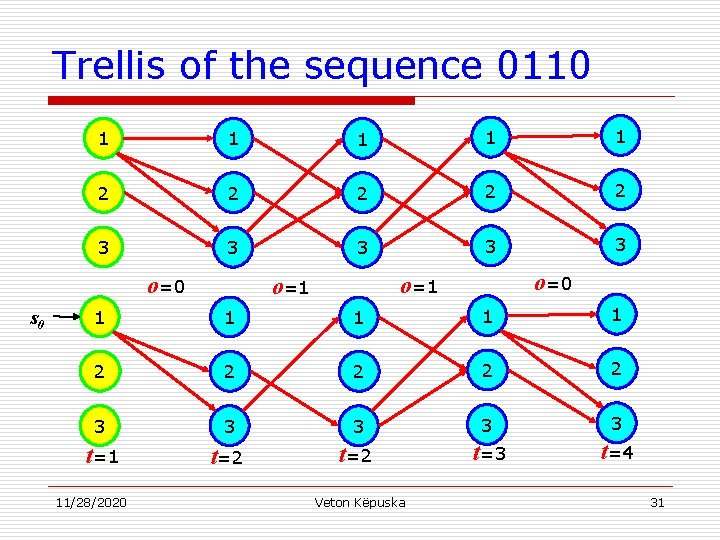Trellis of the sequence 0110 1 1 1 2 2 2 3 3 3 o=0 s 0 o=1 o=1 1 1 2 2 2 3 3 3 t=1 t=2 t=3 t=4 11/28/2020 Veton Këpuska 31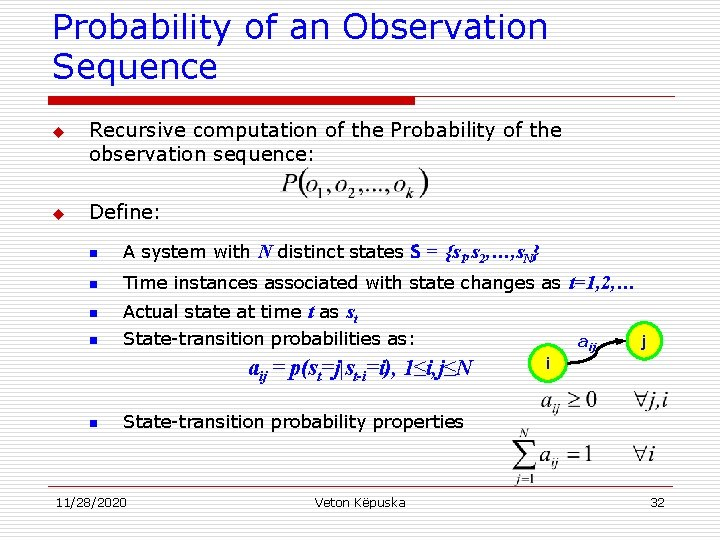Probability of an Observation Sequence u u Recursive computation of the Probability of the observation sequence: Define: n A system with N distinct states S = {s 1, s 2, …, s. N} n Time instances associated with state changes as t=1, 2, … n n Actual state at time t as st State-transition probabilities as: aij = p(st=j|st-i=i), 1≤i, j≤N n aij j i State-transition probability properties 11/28/2020 Veton Këpuska 32Computation of P(O|λ) u Wish to calculate the probability of the observation sequence, O={o 1, o 2, . . . , o. T} given the model . The most straight forward way is through enumeration of every possible state sequence of length T (the number of observations). Thus there are NT such state sequences: u Where: u 11/28/2020 Veton Këpuska 33Computation of P(O|λ) u u Consider the fixed state sequence: Q= q 1 q 2. . . q. T The probability of the observation sequence O given the state sequence, assuming statistical independence of observations, is: Thus: The probability of such a state sequence Q can be written as: 11/28/2020 Veton Këpuska 34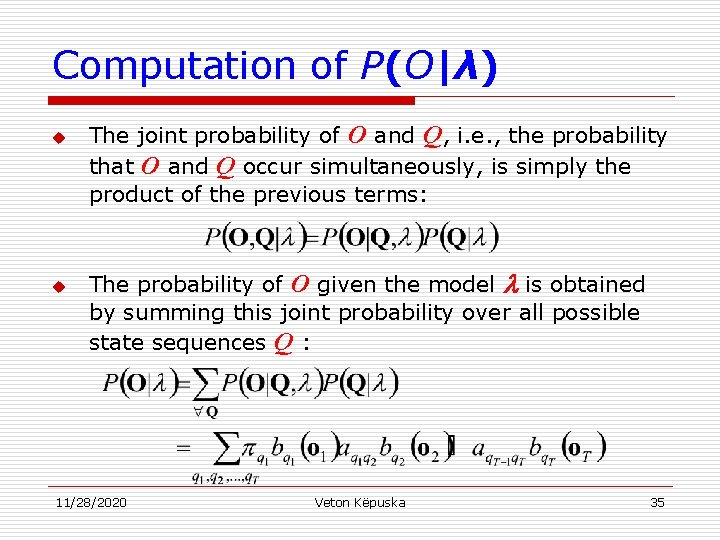Computation of P(O|λ) u u The joint probability of O and Q, i. e. , the probability that O and Q occur simultaneously, is simply the product of the previous terms: The probability of O given the model is obtained by summing this joint probability over all possible state sequences Q : 11/28/2020 Veton Këpuska 35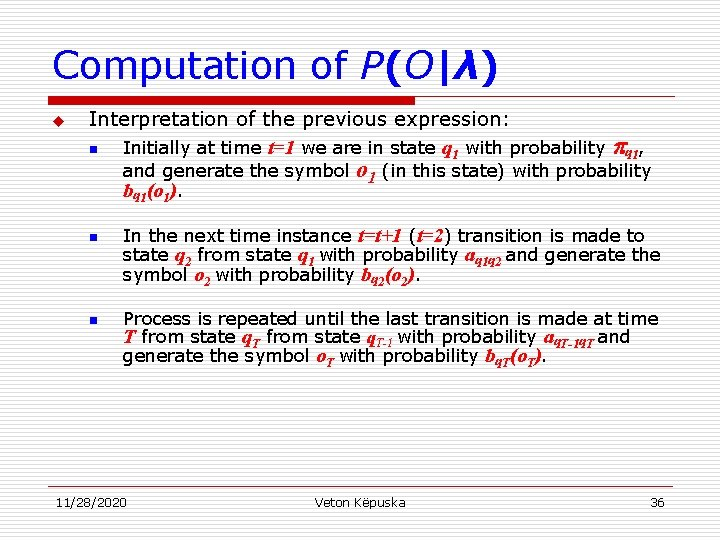Computation of P(O|λ) u Interpretation of the previous expression: n Initially at time t=1 we are in state q 1 with probability and generate the symbol bq 1(o 1). n n q 1, o 1 (in this state) with probability In the next time instance t=t+1 (t=2) transition is made to state q 2 from state q 1 with probability aq 1 q 2 and generate the symbol o 2 with probability bq 2(o 2). Process is repeated until the last transition is made at time T from state q. T-1 with probability aq. T-1 q. T and generate the symbol o. T with probability bq. T(o. T). 11/28/2020 Veton Këpuska 36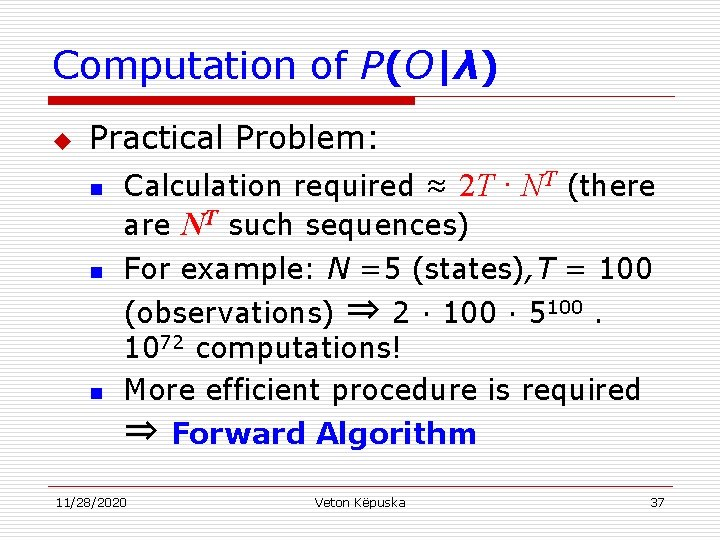Computation of P(O|λ) u Practical Problem: n Calculation required ≈ 2 T · NT (there are NT such sequences) For example: N =5 (states), T = 100 n (observations) ⇒ 2 · 100 · 5100. 1072 computations! More efficient procedure is required n ⇒ Forward Algorithm 11/28/2020 Veton Këpuska 37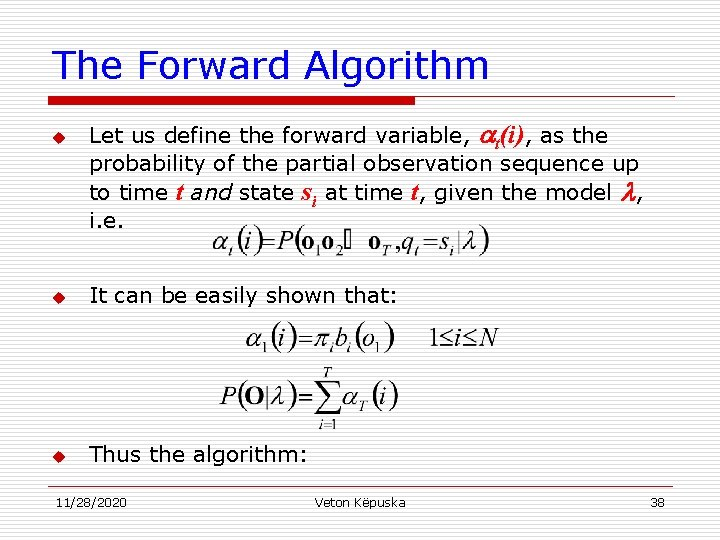The Forward Algorithm u Let us define the forward variable, t(i), as the probability of the partial observation sequence up to time t and state si at time t, given the model , i. e. u It can be easily shown that: u Thus the algorithm: 11/28/2020 Veton Këpuska 38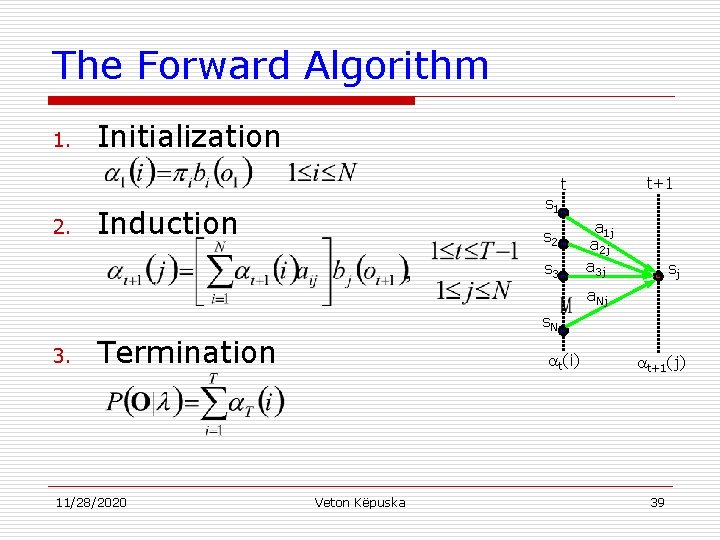The Forward Algorithm 1. Initialization t+1 t 2. s 1 Induction s 2 s 3 a 1 j a 2 j a 3 j sj a. Nj s. N 3. Termination 11/28/2020 t(i) Veton Këpuska t+1(j) 39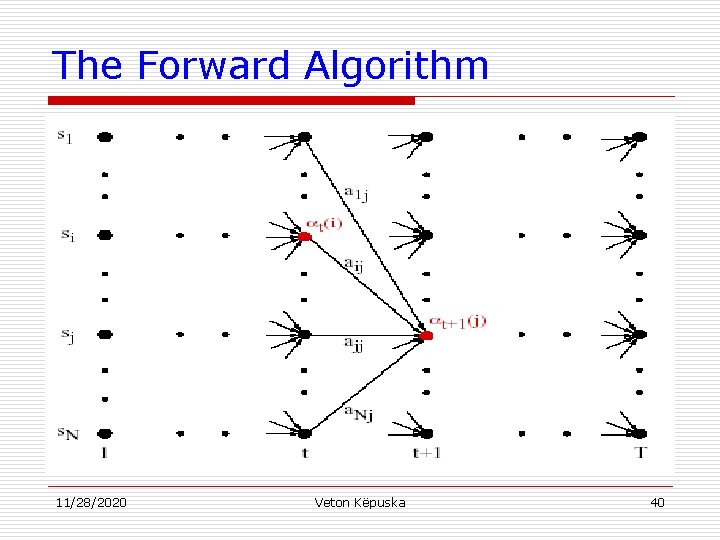The Forward Algorithm 11/28/2020 Veton Këpuska 40